# ISNONTEXT Function

Checks if a cell contains text or not

## What is the ISNONTEXT Function?

The ISNONTEXT Function is categorized under Excel Information functions. The function helps to check if a cell contains text or not (is anything typed into a cell?). It will return TRUE if the value is text and if not, FALSE. For example, if the given value is a number, date, or time, ISNONTEXT will return a FALSE.

As a financial analyst, when dealing with large amounts of data, the function helps in testing if a given value is a text or not. It is also useful in some other scenarios. For example, you performed AUTOSUM for a large amount of data. When the result gives you an error, we can check if there is text in between (or not).

### Formula

=ISNONTEXT(value)

The ISNONTEXT function uses the following arguments:

1. Value (required argument) – This is the expression or value that needs to be tested. It is generally provided as a cell address.

The ISNONTEXT function will return a logical value, which is TRUE or FALSE.

### How to use the ISNONTEXT Function in Excel?

To understand the uses of the ISNONTEXT function, let’s consider a few examples:

#### Example 1

Let’s first understand how the function behaves when given the following set of data:

DataFormula givenResultRemarks
TRUE=ISNONTEXT(TRUE)FALSEThe value is a text, so the function returned FALSE.
=ISNONTEXT( )TRUEThe function returned TRUE for blank values.
“CFA”=ISNONTEXT(“CFA”)TRUEAlthough the expression is text as it was in inverted commas, the function returned TRUE.
#NAME?=ISNONTEXT(#NAME?)TRUEThe function returned TRUE for formula errors.
32.00=ISNONTEXT(32)TRUEThe function returned TRUE as it is a number.

#### Example 2

Suppose we imported data from a PDF file. The data is a set of numbers from 1-20,500, which we imported into column A, as shown below: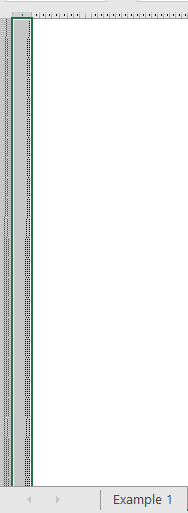As you can see above, the data was zoomed to selection so it’s not understandable. We wish to do a summation of the numbers. When we did that, we got a sum that was different from what we are expecting. While going through the data, we noticed two things:

1. First, the number of rows filled with data is 20,529, whereas the data imported should show 20,500 rows filled.
2. While going through the data, we realized that there are a few rows that contain text.

First, we need to remove the rows that contain text. To do it, we can use ISNONTEXT formula (to see is anything typed into a cell).

In column B, we will enter the formula shown below: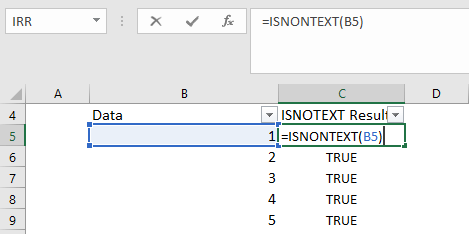Now we will drag the formula until the end to cover all rows.

Once it is done, we will apply a filter. For the filter, we need to go to the DATA tab and click Filter, as shown below: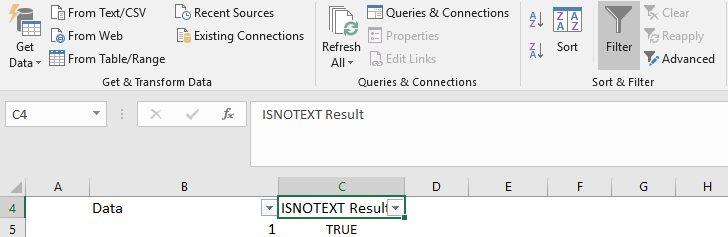After selecting the filters, we can click on the downward arrow and click only on FALSE to get rows with FALSE on them.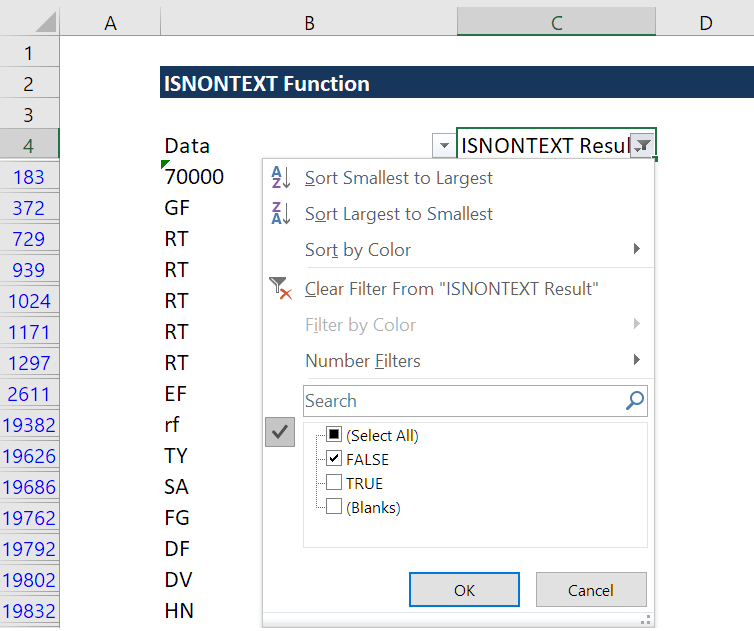The shortcut is ALT+A+T.

Now we see the culprits, which are the rows that contain text. We can now delete them and crosscheck with the original data. Here, there are 26 rows with text, meaning one row was imported as text. We can even correct the error and format it to a number.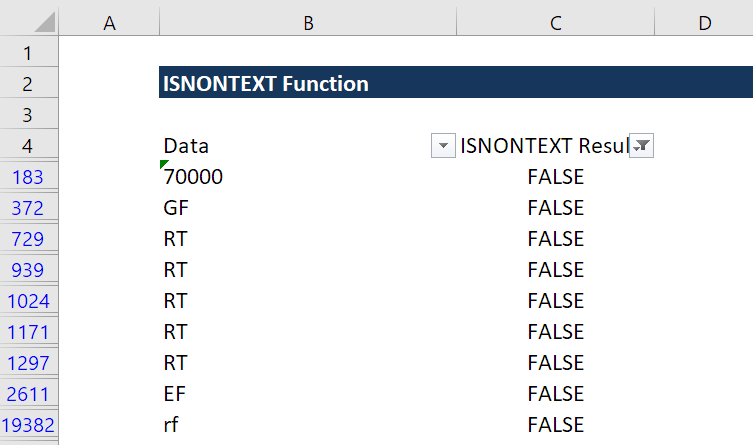### A few notes about the ISNONTEXT Function

1. The ISNONTEXT function is part of IS functions that return the logical values TRUE or FALSE.
2. It doesn’t return an error such as #NAME!, #N/A!, etc., as it just evaluates data.
3. We can determine, is anything typed into a cell?

Thanks for reading CFI’s guide to important Excel functions! By taking the time to learn and master these functions, you’ll significantly speed up your financial analysis. To learn more, check out these additional CFI resources:

• Excel Functions for Finance